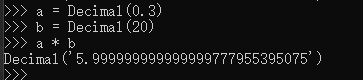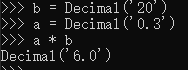• ## python小数

千次阅读 2018-08-31 18:05:10
python小数是通过导入一个模块调用函数后创建的，而不是通过运行常量表达式创建的（运行常量表达式创建的是浮点型）。从功能上来说小数对象就是浮点数，只不过它们有固定的位数和小数点，因此小数是有固定精度的...
目录

一、小数与浮点数

二、小数精度自动升级

三、设置全局精度（高精度）

3.1 什么是精度

3.2 设置高精度（超过17位的精度）

一、小数与浮点数

在python中小数是通过导入一个模块调用函数后创建的，而不是通过运行常量表达式创建的（运行常量表达式创建的是浮点型）。从功能上来说小数对象就是浮点数，只不过它们有固定的位数和小数点，因此小数是有固定精度的浮点值。

浮点数缺乏精确性，因为用来存储数值的空间有限。例如：下面的计算应该得到0，但是却没有，结果接近零，但是却没有足够的位数去实现这样的精度。因为与硬件相关的浮点数运算在精度方面存在缺陷。

a=0.1+0.1+0.1-0.3
print(a)

输出为 5.551115123125783e-17

不过使用小数却可以改变这一缺陷，：

from decimal import  Decimal
c=Decimal('0.1')+Decimal('0.1')+Decimal('0.1')-Decimal('0.3')
print(c) #0.0

二、小数精度自动升级

当有不同精度的小数在表达式中混编时，python自动升级为小数位数最多的：

from decimal import  Decimal
c=Decimal('0.1')+Decimal('0.01')+Decimal('0.05')-Decimal('0.0093')
print(c) #0.1507，自动升级为四位小数

三、设置全局精度（高精度）

3.1 什么是精度

浮点数是用机器上浮点数的本机双精度(64 bit)表示的。提供大约17位的精度和范围从-308到308的指数。和C语言里面的double类型相同。Python不支持32bit的单精度浮点数。如果程序需要精确控制区间和数字精度，可以考虑使用numpy扩展库。

Python 3.X对于浮点数默认的是提供17位数字的精度。

关于单精度和双精度的通俗解释：

单精度型和双精度型，其类型说明符为float 单精度说明符，double 双精度说明符。在Turbo C中单精度型占4个字节（32位）内存空间，其数值范围为3.4E-38～3.4E+38，只能提供七位有效数字。双精度型占8 个字节（64位）内存空间，其数值范围为1.7E-308～1.7E+308，可提供16位有效数字。

3.2 设置高精度（超过17位的精度）

使用decimal模块中的getcontext()函数，先看一下这个函数：

import  decimal
print(decimal.getcontext())

输出为：

Context(prec=28, rounding=ROUND_HALF_EVEN, Emin=-999999, Emax=999999, capitals=1, clamp=0, flags=[], traps=[InvalidOperation, DivisionByZero, Overflow]）

从输出结果中可以看出默认的精度是28位，但是可以设置为更高。这样在分析复杂的浮点数的时候，可以有更高的自己可以控制的精度。其实可以留意下context里面的这rounding=ROUND_HALF_EVEN 参数。ROUND_HALF_EVEN， 当half的时候，靠近even.

import  decimal
print(decimal.Decimal(1)/decimal.Decimal(7)) #0.1428571428571428571428571429
decimal.getcontext().prec=4
print(decimal.Decimal(1)/decimal.Decimal(7)) #0.1429
c=Decimal('0.1')+Decimal('0.01')+Decimal('0.05')-Decimal('0.00593')
print(c) #0.1541

也可以处理50位精度的小数：

import  decimal
print(decimal.Decimal(1)/decimal.Decimal(7)) #0.1428571428571428571428571429
decimal.getcontext().prec=50
print(decimal.Decimal(1)/decimal.Decimal(7))

输出为：

0.14285714285714285714285714285714285714285714285714


展开全文• python进行运算时，如果会出现浮点数，有时候会需要对小数精度进行控制，主要方法有以下几种：1、round（）round()是python的内置方法， round()如果只有一个数作为参数，不指定位数的时候，返回的是一个整数，...
用python进行运算时，如果会出现浮点数，有时候会需要对小数精度进行控制，主要方法有以下几种：

1、round（）

round()是python的内置方法， round()如果只有一个数作为参数，不指定位数的时候，返回的是一个整数，而且是最靠近的偶数整数(python2.7版本则是四舍五入，这个针对的是python3版本）。如果有两个参数则最多保留精度为第二个参数大小。

In : 4 - 3.6
Out: 0.3999999999999999

In : round(4 - 3.6)
Out: 0.0

In : round(4-3.6,2)
Out: 0.4

In : round(4-3.6,5)
Out: 0.4

In : round(2.55555,3)
Out: 2.556

In : round(2.555,30)
Out: 2.555

In : round(2.5)  #注意这个从python3开始是向偶数看齐，即整数部分为偶数时舍弃小数部分，为奇数时进一位
Out: 3.0  #python3输出为2

In : round(3.5)
Out: 4.0

python文档说明如下：
round(number[, ndigits])

Return the floating point value number rounded to ndigits digits after the decimal point. If ndigits is omitted, it defaults to zero. The result is a floating point number. Values are rounded to the closest multiple of 10 to the power minus ndigits; if two multiples are equally close, rounding is done away from 0 (so, for example, round(0.5) is 1.0 and round(-0.5) is -1.0).

Note

The behavior of round() for floats can be surprising: for example, round(2.675, 2) gives 2.67 instead of the expected 2.68. This is not a bug: it’s a result of the fact that most decimal fractions can’t be represented exactly as a float. See Floating Point Arithmetic: Issues and Limitations for more information.


注意：round第二个参数可以为负数，这是用在整数位四舍五入的时候：

In : round(12345, -1)
Out: 12340

In : round(12345, -2)
Out: 12300

In : round(12345, -3)
Out: 12000

In : round(15689, -4)
Out: 20000

In : round(15689, -3)
Out: 16000

In : round(15689.3, -3)
Out: 16000.0


2、使用格式化

In : '%.2f'%3.1415926
Out: '3.14'

In : float('%.3f'%1.234567890)
Out: 1.235

Python默认的是17位精度,也就是小数点后16位，但是这里有一个问题，就是当我们的计算需要使用更高的精度（超过16位小数）的时候该怎么做呢？

方法1：继续像上面使用格式化

In : '%.30f'%(1.0/3)
Out: '0.333333333333333314829616256247'

方法2：使用decimal模块，配合使用其中的getcontext

In : from decimal import Decimal, getcontext

In : Decimal(1) / Decimal(3)
Out: Decimal('0.3333333333333333333333333333')

In : getcontext().prec = 10

In : Decimal(1) / Decimal(3)
Out: Decimal('0.3333333333')

In : len(str(_))
Out: 12  #有2个是前面的0和小数点，所以恰好是10位

In : print getcontext()
Context(prec=10, rounding=ROUND_HALF_EVEN, Emin=-999999999, Emax=999999999, capitals=1, flags=[Inexact, Rounded], traps=[DivisionByZero, Overflow, InvalidOperation])


In : from decimal import localcontext

In : a = Decimal('1.3')

In : b = Decimal('1.7')

In : a / b
Out: Decimal('0.7647058823529411764705882353')

In : print (a/b)
0.7647058823529411764705882353

In : with localcontext() as ctx:
...:     ctx.prec = 3
...:     print (a/b)
...:
0.765

In : with localcontext() as ctx:
...:     ctx.prec = 50
...:     print (a/b)
...:
0.76470588235294117647058823529411764705882352941176

具体见：https://docs.python.org/2.7/library/decimal.html#module-decimal

另：注意下这种情况：

In : nums = [1.23e+18, 1, -1.23e+18]

In : sum(nums)  #注意这个，输出根本不是预想中的那样
Out: 0.0

此时需要math模块：

In : import math

In : math.fsum(nums)
Out: 1.0


In : math.fsum?
Docstring:
fsum(iterable)

Return an accurate floating point sum of values in the iterable.
Assumes IEEE-754 floating point arithmetic.
Type:      builtin_function_or_method

原理详解部分：

我们知道，将一个小数转化为二进制表示的方式是，不断的乘2，取其中的整数部分。例如

(1) 0.625*2 = 1.25, 整数部分为1，小数部分为0.25
(2) 0.25 * 2 = 0.5 , 整数部分为0，小数部分为0.5
(3) 0.5 * 2 = 1 , 整数部分为1，小数部分为0

所以0.625的二进制表示就是0.101。

然而有些小数，例如0.4，并不能够精确的转化为二进制表示，用上面的这种方法计算：

(1) 0.4*2=0.8 整数部分为0，小数部分为0.8
(2) 0.8*2=1.6 整数部分为1，小数部分为0.6
(3) 0.6*2=1.2 整数部分为1，小数部分为0.2
(4) 0.2*2=0.4 整数部分为0，小数部分为0.4

(5) 0.4*2=0.8 整数部分为0，小数部分为0.8
(6) 0.8*2=1.6 整数部分为1，小数部分为0.6
(7) 0.6*2=1.2 整数部分为1，小数部分为0.2
……

所以0.4转化为二进制，应该是0.0110… 这样一个无限循环小数。

计算机的内存、cpu寄存器等等这些硬件单元都是有限的，只能表示有限位数的二进制位，因此存储的二进制小数就会和实际转换而成的二进制数有一定的误差。（你可以试着将0.3转化为二进制表示，也将出现一个循环小数。）

实际上，大多数情况下，小数在计算机中是以一种类似科学计数法的形式表示的，具体的可以参考一下其他的资料。但即便如此，仍然存在误差。

浮点数是用机器上浮点数的本机双精度(64 bit)表示的。提供大约17位的精度和范围从-308到308的指数。和C语言里面的double类型相同。Python不支持32bit的单精度浮点数。所以在python中不建议直接将两个浮点数进行大小比较，或者做精确的计算，往往会得到意想不到的结果。当然，如果非要用，可以参考decimal模块的相关内容。如果程序需要精确控制区间和数字精度，可以考虑使用numpy扩展库。
另外记录个链接：http://justjavac.com/codepuzzle/2012/11/11/codepuzzle-float-who-stole-your-accuracy.html

参考：https://www.zhihu.com/question/25457573/answer/30851062
展开全文decimal getcontext Decimal
• 如图直接把数字加载到decimal.Decimal类里进行乘法运算会出现不精确的情况. 改为字符串赋值进去就好了.如图直接把数字加载到decimal.Decimal类里进行乘法运算会出现不精确的情况.改为字符串赋值进去就好了.


展开全文• 1、基本操作符1）+、-、*和/其中，1/2的结果是0，在python2中/表示整除，python3中使用//进行整除。2）那么除不尽时，整数和小数怎么表示：1.0/2的结果时0.52、另外的操作符1）指数——幂 **3**5，表示5个3相乘；2...
1、基本操作符1）+、-、*和/其中，1/2的结果是0，在python2中/表示整除，python3中使用//进行整除。2）那么除不尽时，整数和小数怎么表示：1.0/2的结果时0.52、另外的操作符1）指数——幂   **3**5，表示5个3相乘；2）取余%3%5的余数是2
展开全文• python的加减乘除： 加：a = b + c 减：a = b - c 乘：a = b * c 除：a = b / c (此处与java不同，这里求出来的为小数，并不是整数位) 求余：a = b % c 次方：a = b ** c (b的c次方)...
• 环境：python3.x a,b = 60,164 一、算数运算符 操作符 描述 例子 + 加法 a+b = 224 - 减法 a-b = -104 * 乘法 a*b = 9840 / 除(保留小数位) 60/2 = 30.0 // 整除 ...
• 原因解释： 浮点数（小数）在...python是以双精度（64bit）来保存浮点数的，后面多余的会被砍掉，所以在电脑上实际保存的已经小于0.1的值了，后面拿来参与运算就产生了误差。 解决办法： 使用decimal库 from decim
• 从统计学的角度，“奇进偶舍”比“四舍五入”更为精确：在大量运算时，因为舍入后的结果有的变大，有的变小，更使舍入后的结果误差均值趋于零。而不是像四舍五入那样逢五就进位，导致结果偏向大数，使得误差产生积累...
• \求方 pow(x,y) ...math.modf 返回整数和小数部分 math.sqrt 开方 import random 封装随机库 print(random.choice([1,2,3,4,5,6,7,8,9])) 取随机数 print(random.choice(range(6))) 等价于 print (rand
• 0.00011001100110011001100110011001100110011001100110011001100python是以双精度（64bit）来保存浮点数的，后面多余的会被砍掉，所以在电脑上实际保存的已经小于0.1的值了，后面拿来参与运算就产生了误差。...
• ## python的运算符号

千次阅读 2016-04-10 19:28:11
，以执行地板除：//除法不管操作数为何种数值类型，总是会舍去小数部分，返回数字序列中比真正的商小的最接近的数字。 >>>1//2 0 >>>1.0//2 0 >>>-1//2.0 -1   模除：% 绝对值：abs() abs(-5)=5 求
• Demo01 ''' 数据：华氏温度 摄氏温度 指令： 1、提示用户输入华氏温度 2、将华氏温度转换摄氏温度 ...#.0保留0位小数、 .1保留一位小数 Demo02 ''' 数据：圆柱高、底面圆半径 指令： 1、提示输入：高、半
• 算术运算 + - * / / ——小数除法 10/2 = 5.0 // ——小数得整数部分 11/2 = 5 % ——取余数 7%3 = 3 关系运算 < > >= <= == != 5>=5 ——True 5==5 ——True 3! =4 ——True 逻辑运算 结果——True ...
• 例如，十进制小数部分0.125具有1/10 + 2/100 + 5/1000的值。同样，二进制小数 0.001具有值0/2 + 0/4 + 1/8。 这两个小数具有“相同的值”，唯一真正的区别是第一个分数用10进制表示，第二个用2进制表示。 不幸的是...
• Python3进行除法运算，如果想丢弃小数部分，即执行整数运算，可使用双斜杠。 　示例： 　>>>1 // 2 　>>>0 python3求余运算符，x % y的结果位X除以Y的余数。换而言之执行整除时余下的部分，即...
• 菜鸟教程-Python3-Python数字 ...结果均返回几位小数运算结果，尽可能地保留 3-在交互模式中，最后被输出的表达式结果被赋值给变量 _ 默认保留在下划线里，然后可以调用时使用这个结果 另外，_ 变量...
• 这是因为 在python的浮点数运算***存在不确定尾数***的关系。 ------round(x,d):对x四舍五入,d是小数截取位数 ------不确定尾数一般发生在10的-16左右，round()十分有效 举例： ...
• Python的数值运算基本...注：python中整数除整数除不开时用小数表示（与java不同） 取余 10%3 运行结果 1 （）运算 2*(1+2) 运行结果 6 次方（**） 2**3 #二的三次方 运行结果 8 基本运算的顺序：同数学中的
• 2019独角兽企业重金招聘Python工程师标准>>> ...
• 2019独角兽企业重金招聘Python工程师标准>>> ...
• 运算符 1.赋值运算符 1）“=”赋值运算 先算右边表达式内的运算，放入左边 ...# // 整除，会直接去掉结果的小数部分，结果是正整数 div1 = 6.5 // 2.3 print(div1) #求余的结果是正负号总是保持一致 mod = 23......

# python小数运算python 订阅